Reliability /Safety Index Page
Statistics Index Page

Failure Distributions

Note: The information below is provided as an overview of failure distributions.. More detailed and reliable information is provided at the sites linked at the bottom of this page...

Introduction

In determining the lifetime reliability of a population of components (bearings, seals, gears etc.) sample information is obtained from testing programmes and operational feedback on the failure history of components belonging to the population.  From the information obtained it is possible to produce a graph of the probability density function f(t).   This is a plot of the frequency at which components fail as a function of time divided by the whole population.

The pdf function has the property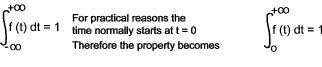Associated with the pdf is the Cumulative Density Function F(t).  This is simply a plot of the cumulative fraction of the failure population against time.  It is the integral of the f(t) against time (t).

The CDF has the properties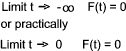This effectively means that at time 0 no failures have occurred.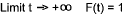At infinity the whole population of components will have failed.

Reliability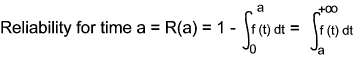The reliability may be expressed that.. for time = a ( e.g 10 years ) there is a chance of the item surviving (not failing)... = 1 in 10 is likely to fail.

Hazard Rate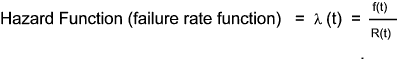The hazard rate may be expressed as... the failure rate will be 2 x 10 -4 (failures /unit time) or 2 failures per 10 4 time units

Mean Life Function

The mean life provides the average life to failure of components is also called the Mean Life Between Failures (MLBF) and the Mean Time to Failures (MTTF)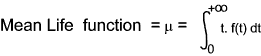The MTTF /MTBF may be expressed as say 1,000 hours at which 50% of units have failed

Failure Distributions

The pdf curve can take many forms....Some of the different distributions are listed below

Normal Distribution

One curve representing purely random events is the normal (gaussian) curve.
This is shown below with the associated CDF.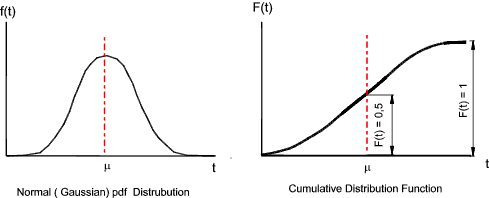The equation for the normal distribution is :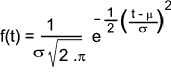μ = The mean (MTTF , MTBF) σ = The standard Deviation

Both of these parameters are estimated from the data, i.e. the mean and standard deviation of the data.   From these parameters f(t) is fully defined enabling evaluation of f(t) from any value of t.

Note: The standard deviation is a measure of scatter of the information.    A small standard deviation is a thinner higher bell and a large standard deviation is a wider flatter bell.

Normal Distributions are appropriate in the following conditions

 There is a strong tendency for the variable to take a central value; Positive and negative deviations from this central value are equally likely; The frequency of deviations falls off rapidly as the deviations become larger.

The Lognormal Distribution

The lognormal distribution is commonly used for general reliability analysis, cycles to failure in fatigue and material strengths and loading.

The data follows the lognormal distribution when the natural logarithms of the times-to-failure are normally distributed.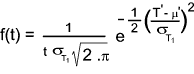• σT1 = Standard Deviations of the natural logarithm of times-to-failure
• μ' = Mean of the natural logarithm of times-to-failure

Weibull Distribution

The Weibull distribution is a general-purpose reliability distribution used to model material strength, times-to-failure of electronic and mechanical components, equipment, or systems.   In its most general case, the three-parameter Weibull pdf is defined by: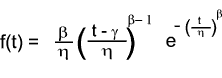with three parameters, where :

 β = shape parameter γ = location parameter η = scale parameter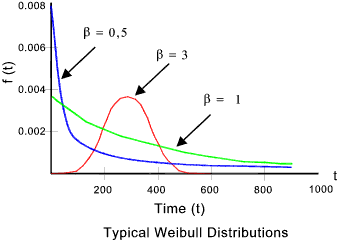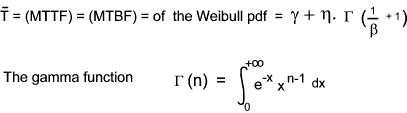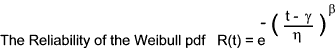If the location parameter γ is assumed to be zero then the distribution is known as the two-parameter Weibull distribution...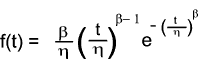The β = shape parameter gives indications on the prevalent failure modes.

 β < 1 indicates 'infant mortality' due to poor production quality or insufficient burn-in β = 1 indicates random failures which are independent of time. Human errors , natural events etc. β = 1 to 4 indicates early wear out i.e. erosion, corrosion, early fatigue 1 β > 4 indicates old age and rapid wear out. bearing failures, corrosion, erosion, fatigue etc.

The Exponential Distribution

The exponential distribution is a commonly used distribution in reliability engineering.   Mathematically, it is a fairly simple distribution, which sometimes leads to its use in inappropriate situations.   This distribution is used to model the behavior of units that have a constant failure rate.

An exponential distribution can easily be described as follows...
If a thousand items have a constant failure rate of 10% per month.  After the first month 100 items have failed (0.1 x 1000) leaving 900 items.  After the second month 90 items will have failed (0.1 x 900) leaving 810 items...  After 12 months 31 items will fail leaving 282 items.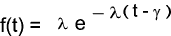λ = Scaling factor=Failure Rate γ = Location factor

The mean time to failure of this distribution is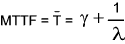If the location parameter γ is assumed to be zero then the distribution is called the one parameter exponential distribution.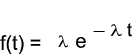The mean time to failure and the reliability of this distribution is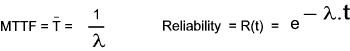Links to Failure Distributions Baringer1 ...Reliability Links and Information Weibull ...Reliability analysis articles and software Reliability Magazine ...Magazine devoted to reliability Weibull Databases ...Very useful reference information SRC ...Center of Reliability and Maintainability . An Useful reference site Reliability Direct ...Reliability and Condition products. ACUSAFE ...An internet resource for Safety and Risk Management.

Reliability /Safety Index Page
Statistics Index Page